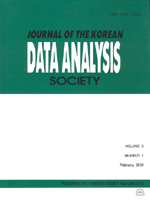상세검색
최근 검색어 전체 삭제
다국어입력
즐겨찾기0KCI등재 학술저널

# Behavior of local polynomial regression estimators at boundary point

• 등재여부 : KCI등재
• 2005.04
• 419 - 427 (9 pages)

The asymptotic theory has played an important role in the smoothing literature over the past 20 years. However, it is relatively well known that the asymptotic results do not guarantee to hold up for the finite sample case. In this paper, we deal with a little bit different problem of the asymptotic result. When we make a comparison between methods in terms of the asymptotic result, we usually concentrate our attention on the rate of convergence. The rate of convergence tells us the story about the estimator when we have infinitely large sample. In practice, however, we never encounter such case. Therefore, we need to look at the asymptotic expression more extensively. In this paper, we compare the leading terms of the asymptotic MSE of the local constant estimator and the local linear estimator at the boundary point, and find out the conditions on which local constant estimator has the smaller asymptotic MSE than that of local linear estimator at the boundary point.

1. Introduction

2. Comparison

3. Discussions

Reference